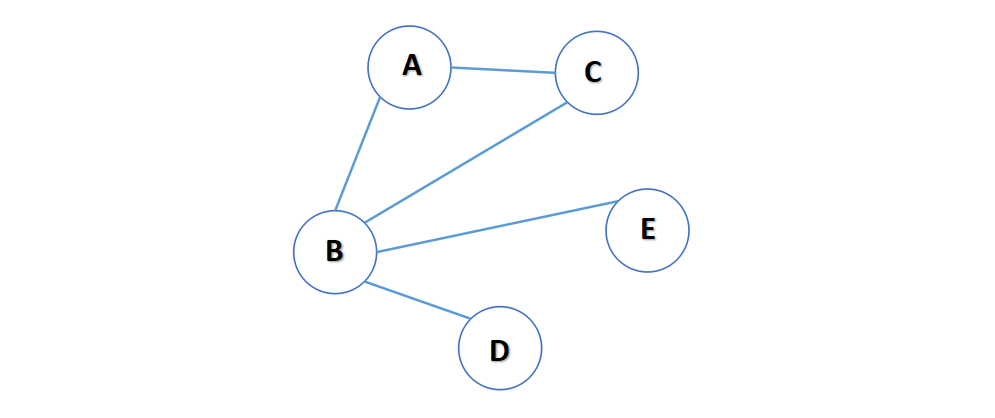# 图的介绍# 图的表现形式# 代码实现简易图

## 成员变量

public class Graph {
private ArrayList<String> vertexList; // 存储顶点集合
private int[][] edges; // 存储图对应的邻接矩阵
private int numOfEdges; // 边的数目
}


## 基本方法编写

### 构造方法

public Graph(int n) {
// 初始化矩阵和vertexList
edges = new int[n][n];
vertexList = new ArrayList<>();
numOfEdges = 0;
}


### 添加节点和添加边

// 添加节点
public void insertVertex(String vertex) {
}
// 添加边
/**
* @param v1     第一个点的下标
* @param v2     第二个顶点的下标
* @param weight 表示权值
*/
public void insertEdge(int v1, int v2, int weight) {
edges[v1][v2] = weight;
edges[v2][v1] = weight;
numOfEdges++;
}


### 其余方法

// 返回节点的个数
public int getNumOfVertex() {
return vertexList.size();
}
// 得到边的数目
public int getNumOfEdges() {
return numOfEdges;
}
// 返回节点i对应的数据
public String getValueByIndex(int i) {
return vertexList.get(i);
}
// 显示图对应的矩阵的方法
public void showGraph() {
for (int[] link : edges) {
}
}


# 图的遍历

## 准备工作

// 得到第一个邻接节点的下标w
public int getFirstNeighbor(int index) {
for (int j = 0; j < vertexList.size(); j++) {
if (edges[index][j] > 0) {
return j;
}
}
return -1;
}
// 根据前一个邻接节点的下标来获取下一个邻接节点
public int getNextNeighbor(int v1, int v2) {
for (int j = v2 + 1; j < vertexList.size(); j++) {
if (edges[v1][j] > 0) {
return j;
}
}
return -1;
}


## 深度优先遍历(DFS)

1. 从初始访问节点出发，先访问第一个邻接节点，然后将第一个邻接节点再作为初始访问节点继续访问它的第一个邻接节点。
2. 很显然这个思想就是向深度挖掘而不是先遍历完所有的邻接节点。
3. 这个过程是个递归的过程

1. 访问初始节点v，并将v置为已访问
2. 查找v的第一个邻接节点w
3. 若w存在，继续执行4，若v不存在则对v的下一个节点继续
4. w没被访问过，对w进行深度优先遍历
5. 查找v的第二个邻接节点继续第3步
// 对dfs进行重载
public void dfs() {
isVisited = new boolean[getNumOfVertex()];
for (int i = 0; i < getNumOfVertex(); i++) {
if (!isVisited[i]) {
dfs(isVisited, i);
}
}
}
// 对节点v进行深度优先遍历
public void dfs(boolean[] isVisited, int i) {
// 首先我们访问该节点，输出
System.out.print(getValueByIndex(i) + "->");
// 将节点设置为已经访问
isVisited[i] = true;
// 获取节点i的第一个邻接节点
int w = getFirstNeighbor(i);
while (w != -1) { // 说明有
if (!isVisited[w]) {
dfs(isVisited, w);
}
// w被访问过
w = getNextNeighbor(i, w);
}
}


## 广度优先遍历

1. 访问初始节点v，标记v已访问
2. 节点v入队列
3. 当队列非空时继续执行，否则算法结束。
4. 出队列取得对头节点u
5. 查找节点u的第一个邻接节点w
6. 若节点u的邻接节点w不存在，则转到步骤3，否则循环以下3步骤
1. 若w未被访问，则访问节点w并标记为访问
2. 节点w入队列
3. 查找节点u的后继w邻接节点后的下一个节点w，转到步骤6
// 对一个节点进行广度优先遍历

public void bfs(boolean[] isVisited, int i) {
int u; // 表示头节点对应的下标
int w; // 表示邻接节点w
// 需要一个队列，节点访问的顺序
// 访问节点，输出信息
System.out.print(getValueByIndex(i));
// 标记为已访问
isVisited[i] = true;
// 将节点加入队列
while (!queue.isEmpty()) {
// 取出队列的头节点下标
u = (Integer)queue.removeFirst();
// 得到u的第一个邻接节点w
w = getFirstNeighbor(u);
while (w != -1) {
// 找到了 是否访问过
if (!isVisited[w]) {
System.out.print(getValueByIndex(w));
// 标记已经访问
isVisited[w] = true;
// 入队
}
// 以u为前驱点找w后面的下一个邻接节点
w = getNextNeighbor(u, w);
}
}
}
// 重写
public void bfs() {
isVisited = new boolean[getNumOfVertex()];
for (int i = 0; i < getNumOfVertex(); i++) {
if (!isVisited[i]) {
bfs(isVisited, i);
}
}
}


Q.E.D.